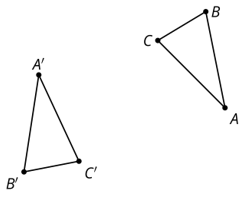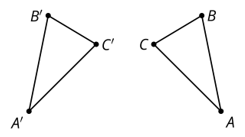# Week 5 Day 2 Lesson SummaryWhich triangle is the original and which is the image?

What would be a sequence of transformations that would take triangle ABC onto triangle A'B'C'? Explain how you know the image of A coincides with A'.

Would that sequence of transformations from above work just for this one pair of congruent triangles, or would it work for other pairs of congruent triangles, too?

What is different about the sequence of transformations for the set of triangles below than from the previous case?What happens when we try the same sequence of transformations from last time?